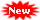### New Update

6/recent/ticker-posts

# 12th Maths Question Bank with Answers Tamil Nadu Plus 2 Maths 1 marks 6 marks & 10 Marks with answers

## 12th Maths Question Bank with Answers Plus 2 Maths 1 mark with Answers 6 mark & 10 marks with answers Tamil Nadu +2 Tamil State Board Maths Question Bank with Answers

### Maths Question Bank with Answers - Part A & Part B Questions with Answers

Rejinpaul.com Provides Question Bank with answers for 12th Standard maths Tamil Nadu. Student can follow the 12th Maths Blue Print given by Tamil Nadu Education Board for preparing for the exams.

Total marks for Tamil will be for 200 marks and time allocated is 3 hours. It consist of Three different sections. Proper weightage for  each and every unit in maths is given in maths Blue Print. 12th Maths Public Question Paper also follows the same.

12th maths Question Bank with Answers

12th Maths Question Bank with answers (ENGLISH MEDIUM)

12th Maths 1 mark Question with answers (ENGLISH MEDIUM)

12th Maths 10 mark Question with answers (ENGLISH MEDIUM)

12th Maths Useful Material (ENGLISH MEDIUM)Special Study Materials from Rejinpaul Team - Click here

__________________________________________________________________________Search by Students :

12th maths Question Bank with Answers
plus 2 maths Question Bank PDF
plus 2 maths Question Bank with answers
Twelth maths Part A and Part B Questions with Answers
12th maths 1 mark and 6 marks Questions
12th maths study Materials
12th maths Question Bank with answers
12th maths important Questions
12th maths Important Questions with answers

1.2.3.